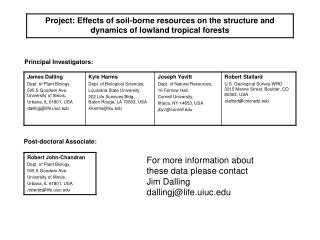DownloadDownload PresentationPrincipal Investigators:

# Principal Investigators:

Télécharger la présentation## Principal Investigators:

- - - - - - - - - - - - - - - - - - - - - - - - - - - E N D - - - - - - - - - - - - - - - - - - - - - - - - - - -
##### Presentation Transcript

2. Acknowledgements Please acknowledge the following agencies that provided funding for the collection and analyses of these data NSF DEB021104,021115, 0212284,0212818 and OISE 0314581 STRI Soils Initiative and CTFS Thanks to Paolo Segre and Juan Di Trani for assistance in the field

3. Table 1. Variogram model fit parameters for soil properties for the BCI 50-ha plot. The measured data values were first transformed using Box-Cox transformation, and then a polynomial trend surface regression was fitted. The residuals from the trend surface regression were used to compute empirical variograms, to which variogram models were fitted. Lambda is the Box-Cox parameter, and trend order indicates the order of the polynomial regression. Effective range for the Exponential variogram model is estimated at 3 times the fitted range because the variogram approaches the sill only asymptotically.

4. Maps: Filled-contour maps plotted using block average data. Aluminum (Al): mg / kg of oven-dried soil

5. Maps: Filled-contour maps plotted using block average data. Boron (B): mg / kg of oven-dried soil

6. Maps: Filled-contour maps plotted using block average data. Calcium (Ca): mg / kg of oven-dried soil

7. Maps: Filled-contour maps plotted using block average data. Copper (Cu): mg / kg of oven-dried soil

8. Maps: Filled-contour maps plotted using block average data. Iron (Fe): mg / kg of oven-dried soil

9. Maps: Filled-contour maps plotted using block average data. Potassium (K): mg / kg of oven-dried soil

10. Maps: Filled-contour maps plotted using block average data. Magnesium (Mg): mg / kg of oven-dried soil

11. Maps: Filled-contour maps plotted using block average data. Manganese (Mn): mg / kg of oven-dried soil

12. Maps: Filled-contour maps plotted using block average data. Nitrogen (N): mg / kg of oven-dried soil

13. Maps: Filled-contour maps plotted using block average data. Nitrogen mineralisation: mg / kg of oven-dried soil

14. Maps: Filled-contour maps plotted using block average data. Phosphorus (P): mg / kg of oven-dried soil

15. Maps: Filled-contour maps plotted using block average data. Zinc (Zn): mg / kg of oven-dried soil